# Convert Date to Julian Format in Excel & Google Sheets

This tutorial will demonstrate how to convert date to Julian Format in Excel & Google Sheets.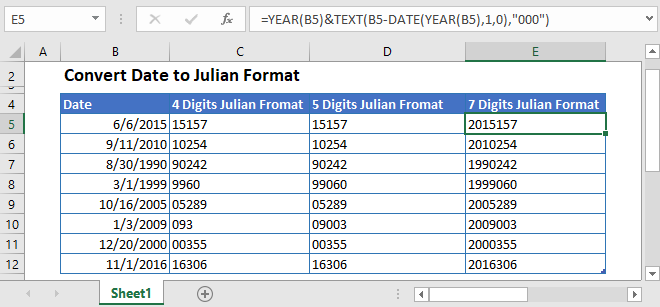There are multiple formulas that can be used to convert date to Julian formats, depending on your exact need. The formulas used for converting a date to Julian Format uses the DATE, YEAR, and TEXT functions.

## What is Julian Date Format

Julian Date Format is referred to as a format which is a combines the current year and the number of the days since the start of the year. For example, December 25, 2010, is represented in Julian Format as 2010359.

Now we got several variations in which Julian Date can be represented. But the most common three variations with their conversion formulas are described below:

## Convert Date to 4 Digits Julian Format

To convert a date into a Julian Format where the first two digits represent the year and the day of the number is represented without padding in the last digits, we use the following formula:

``=TEXT(B3,"yy")&B3-DATE(YEAR(B3),1,0)``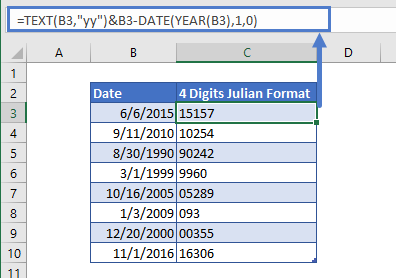The first part of the formula extracts the first two digits that represent the year

``=TEXT(B3,"yy")``

and the second part of the formula returns the last three digits that represents the day of the year

``=B3-DATE(YEAR(B3,1,0)``

Both of these parts are joined together by concatenated with an ampersand (&).

## Convert Date to 5 digits Julian Format

In a five digits number of Julian Format, the first two digits represent the year and the last three digits represent the day of the year. To convert the Excel date to Julian format of five digits, we can do so by using this formula:

``=TEXT(B3,"yy")&TEXT(B3-DATE(YEAR(B3),1,0),"000")``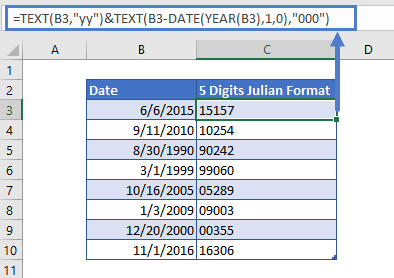The first part of the formula extracts the first two digits that represent the year

``=TEXT(B3,"yy")``

and the second part of the formula returns the last three digits that represents the day of the year

``=TEXT(B3-DATE(YEAR(B3,1,0),"000")``

Both of these parts are joined together by concatenated with an ampersand (&).

## Convert Date to 7 Digits Julian Format

In a seven digits number of Julian Format, the first four digits represent the year and the last three digits represent the day of the year. To convert the Excel date to Julian format of seven digits, we use the following formula:

``=YEAR(B3)&TEXT(B3-DATE(YEAR(B3),1,0),"000")``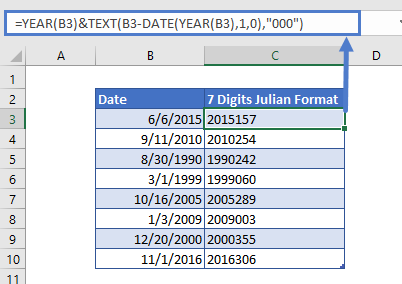The first part of the formula extracts the first two digits that represent the year

``=YEAR(B3)``

and the second part of the formula returns the last three digits that represent the day of the year and the TEXT function is used for padding the day value with zeros,

``=TEXT(B3-DATE(YEAR(B3,1,0),"000")``

Both of these parts are concatenated with an ampersand operator (&).

## Reverse Conversion

If we are given the date in seven digits Julian Format and want to convert it to a simple date, we can use the following formula for conversion:

``=DATE(LEFT(B3,4),1,RIGHT(B3,3))``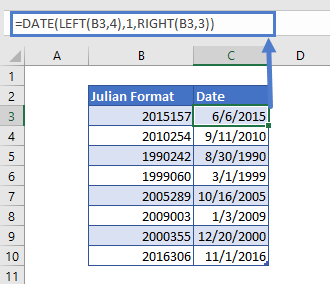## Convert Date to Julian Format in Google Sheets

The conversion formula for Julian Format works exactly the same in Google Sheets as in Excel: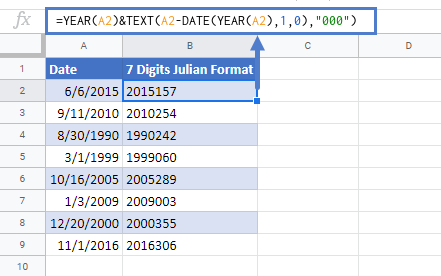### Excel Practice Worksheet

Practice Excel functions and formulas with our 100% free practice worksheets!